# The PANEL Procedure

### Tests for Serial Correlation and Cross-Sectional Effects

Subsections:

The presence of cross-sectional effects causes serial correlation in the errors. Therefore, serial correlation is often tested jointly with cross-sectional effects. Joint and conditional tests for both serial correlation and cross-sectional effects have been covered extensively in the literature.

#### Baltagi and Li Joint LM Test for Serial Correlation and Random Cross-Sectional Effects

Baltagi and Li (1991) derive the LM test statistic, which jointly tests for zero first-order serial correlation and random cross-sectional effects under normality and homoscedasticity. The test statistic is independent of the form of serial correlation, so it can be used with either AR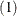or MAerror terms. The null hypothesis is a white noise component: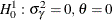for MAwith MA coefficientor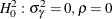for ARwith AR coefficient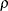. The alternative is either a one-way random-effects model (cross-sectional) or first-order serial correlation ARor MAin errors or both. Under the null hypothesis, the model can be estimated by the pooled estimation (OLS). Denote the residuals as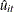. The test statistic is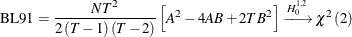where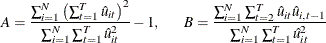#### Wooldridge Test for the Presence of Unobserved Effects

Wooldridge (2002, sec. 10.4.4) suggests a test for the absence of an unobserved effect. Under the null hypothesis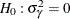, the errors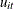are serially uncorrelated. To test, Wooldridge (2002) proposes to test for AR(1) serial correlation. The test statistic that he proposes is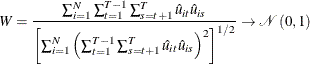whereare the pooled OLS residuals. The test statistic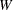can detect many types of serial correlation in the error term u, so it has power against both the one-way random-effects specification and the serial correlation in error terms.

#### Bera, Sosa Escudero, and Yoon Modified Rao’s Score Test in the Presence of Local Misspecification

Bera, Sosa Escudero, and Yoon (2001) point out that the standard specification tests, such as the Honda (1985) test described in the section Honda (1985) and Honda (1991) UMP Test and Moulton and Randolph (1989) SLM Test, are not valid when they test for either cross-sectional random effects or serial correlation without considering the presence of the other effects. They suggest a modified Rao’s score (RS) test. When A and B are defined as in Baltagi and Li (1991) , the test statistic for testing serial correlation under random cross-sectional effects is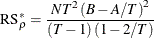Baltagi and Li (1991, 1995) derive the conventional RS test when the cross-sectional random effects is assumed to be absent: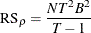Symmetrically, to test for the cross-sectional random effects in the presence of serial correlation, the modified Rao’s score test statistic is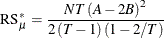and the conventional Rao’s score test statistic is given in Breusch and Pagan (1980). The test statistics are asymptotically distributed as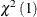.

Because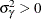, the one-sided test is expected to lead to more powerful tests. The one-sided test can be derived by taking the signed square root of the two-sided statistics: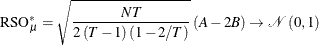#### Baltagi and Li (1995) LM Test for First-Order Correlation under Fixed Effects

Letbe the residual from the fixed one-way model (FIXONE). The two-sided LM test statistic for testing a white noise component in a fixed one-way model (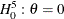or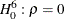, given that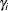are fixed effects) is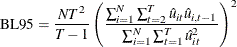The LM test statistic is asymptotically distributed asunder the null hypothesis. The one-sided LM test with alternative hypothesis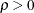is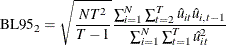which is asymptotically distributed as standard normal.

#### Durbin-Watson Statistic

Bhargava, Franzini, and Narendranathan (1982) propose a test of the null hypothesis of no serial correlationagainst the alternative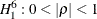by the Durbin-Watson statistic based on residualsfrom the fixed one-way model (FIXONE):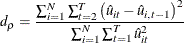The test statistic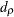is a locally most powerful invariant test in the neighborhood of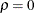. Some of the upper and lower bounds are listed in Bhargava, Franzini, and Narendranathan (1982). For very large N, to test against a positive correlation, you can simply test whether the test statistic d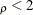.

#### Berenblut-Webb Statistic

Let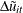be the residuals from the first-difference estimation. Bhargava, Franzini, and Narendranathan (1982) suggest using the Berenblut-Webb statistic, which is a locally most powerful invariant test in the neighborhood of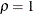. The test statistic is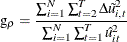The upper and lower bounds are the same as for the Durbin-Watson statistic.

#### Testing for Random Walk Null Hypothesis

You can also use the Durbin-Watson and Berenblut-Webb statistics to test the random walk null hypothesis, with the bounds that are listed in Bhargava, Franzini, and Narendranathan (1982). For more information about these statistics, see the sections Durbin-Watson Statistic and Berenblut-Webb Statistic. Bhargava, Franzini, and Narendranathan (1982) also propose the Rstatistic to test the random walk null hypothesisagainst the stationary alternative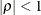. Let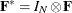, where
is a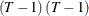symmetric matrix that has the following elements: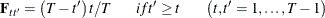The test statistic is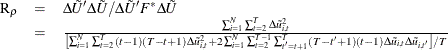The statistics R, g, and dcan be used with the same bounds. They satisfy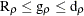, and they are equivalent for large panels.